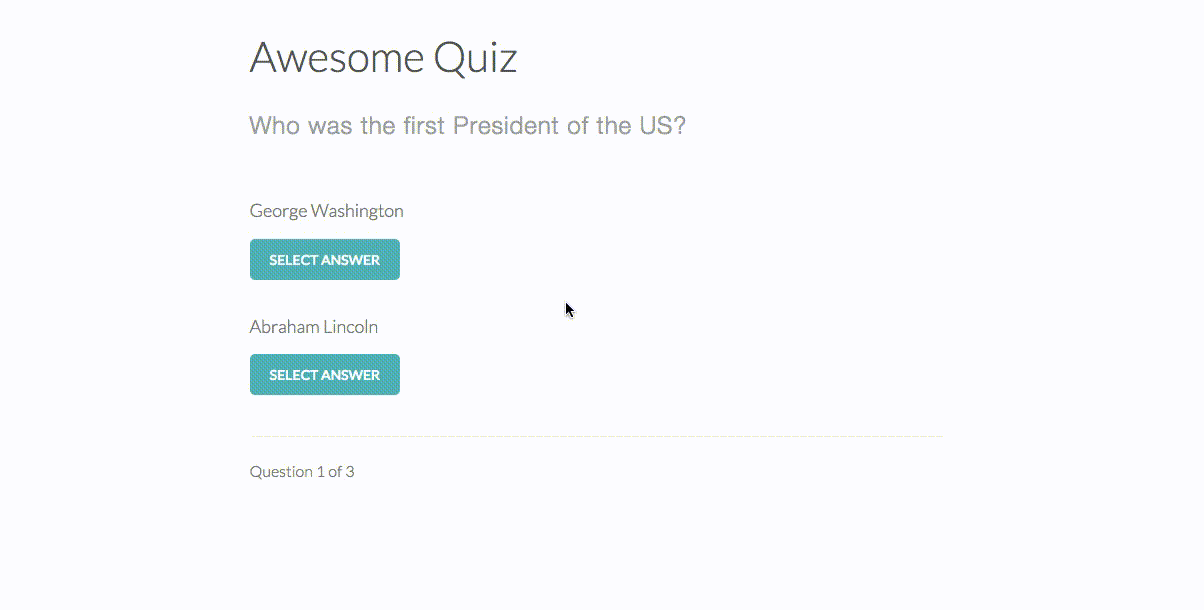# Interactive Quiz Application

As part of the Treehouse Object-Oriented JavaScript course, I built an Interactive Quiz Application that builds a quiz with three questions and two answers, tracks a user’s progress throughout the quiz, and displays their score at the end of the quiz. The index.html and style.css files were provided at the start of the project, but I generated three JavaScript files to make it all come together. You can see the full project including HTML and CSS files on GitHub, but I wanted to walk through some of my thought processes here.

I broke the problem down into several steps:

1. Define an object literal for “Question” and add properties
2. Define an object literal for a “Quiz”
3. Add some initial questions and set the first question to display on page load
4. Track progress and score as users click buttons
5. Display score when quiz questions are finished

### Defining an object literal for “Question” and add properties

This was relatively straightforward. The Question object had four properties – the actual question, choice0, choice1, and the correct answer. Thinking after the fact, I could used an array for the choices. That would have made it easier to add a question with three possible choices in the future.

[code language=”javascript”]
function Question(question, choice0, choice1, correct) {
this.question = question;
this.choice0 = choice0;
this.choice1 = choice1;
this.correct = correct;
}[/code]

### Define an object literal for a “Quiz”

Again, this wasn’t too difficult and only required a few lines of code.

[code language=”javascript”]
// Create object literal of quiz with property of question set to an empty array
function Quiz () {
this.questions = [];
}[/code]

### Add some initial questions and set the first question to display on page load

I defined some new questions in the form of variables using the properties defined in step #1:

[code language=”javascript”]
var president = new Question("Who was the first President of the US?", "George Washington", "Abraham Lincoln", "George Washington");
var cat = new Question("Who is the best cat in the world?", "Garfield", "Denali", "Denali");
var game = new Question("What is the better game?", "Soccer", "Basketball", "Soccer");[/code]

Then, since the Quiz object had a property of questions set to an empty array, I could create a new quiz, define a function that pushed questions onto the empty array, and use that function to add my existing questions. Upon further review, I could’ve done a better job naming the various items. For example, it’s confusing to have a property of “questions” as well as pass “questions” to the function.

[code language=”javascript”]
var quiz = new Quiz();

this.questions.push(questions);
};

Now, I just had to set the initial questions on page load.

[code language=”javascript”]
var question = document.getElementById("question");
var choice0 = document.getElementById("choice0");
var choice1 = document.getElementById("choice1");

question.innerHTML = quiz.questions.question;
choice0.innerHTML = quiz.questions.choice0;
choice1.innerHTML = quiz.questions.choice1;
[/code]

### Track progress and score as users click buttons

Now that the basics were setup, it was time to add some functionality to the quiz so clicking a button actually resulted in progress. First, I setup some initial variables including the buttons users would use to select their answer (guess0 and guess1).

[code language=”javascript”]
var totalCorrect = 0;
var currentQuestion = 0;
var totalQuestions = quiz.questions.length;
var guess0 = document.getElementById("guess0");
var guess1 = document.getElementById("guess1");
[/code]

Next, I setup a function to track a user’s progress throughout the quiz. Since currentQuestion starts at 0, I automatically added one to the variable when tracking progress.

[code language=”javascript”]
// Track progress through quiz
var progress = function () {
var progress = document.getElementById("progress");
progress.innerHTML = "Question " + (currentQuestion+1) + " of " + totalQuestions;
}
[/code]

I defined four additional functions: one that would set the next question, another that incremented totalCorrect, third that tracked when the quiz was actually finished, and a fourth that fired when the quiz was finished.

[code language=”javascript”]
var setQuestion = function (currentQuestion) {
question.innerHTML = quiz.questions[currentQuestion].question;
choice0.innerHTML = quiz.questions[currentQuestion].choice0;
choice1.innerHTML = quiz.questions[currentQuestion].choice1;
}

// Increment totalCorrect when answer is correct
totalCorrect = totalCorrect + 1;
}

// Check to see if this is the last question
var checkDone = function (currentQuestion) {
if (currentQuestion == totalQuestions) {
finish();
} else {
setQuestion(currentQuestion);
progress();
}
}

// Replace quiz with results when finished
var finish = function () {
var quizElement = document.getElementById(‘quiz’);
quizElement.innerHTML = ‘
<h1>Game Over</h1’;
quizElement.innerHTML += ‘
<h2>You got ‘ + totalCorrect + ‘ questions correct.</h2>

‘;
}[/code]

Finally, I added an onclick event to both guess0 and guess1. The event was tied to a function that performed four actions: 1) Check to see if answer is correct. 2) Increment currentQuestion. 3) Increment score counter if correct. 4) Check to see if the quiz was over.

[code language=”javascript”]
// Track results on clicking the first choice
guess0.onclick = function () {
if (quiz.questions[currentQuestion].correct == choice0.innerHTML) {
currentQuestion = currentQuestion + 1;
checkDone(currentQuestion);
} else {
currentQuestion = currentQuestion + 1;
checkDone(currentQuestion);
}
}

// Track results on clicking the second choice
guess1.onclick = function () {
if (quiz.questions[currentQuestion].correct == choice1.innerHTML) {
currentQuestion = currentQuestion + 1;
checkDone(currentQuestion);
} else {
currentQuestion = currentQuestion + 1;
checkDone(currentQuestion);
}
}
[/code]

Finally, I fired the progress function right off the bat to set the initial progress.

[code language=”javascript”]
// Set initial progress 0 of total questions
progress();[/code]

Here’s the finished product:Strategies on solving problems and wowing customers every Sunday 👉
Strategies for solving problems and wowing customers 👇